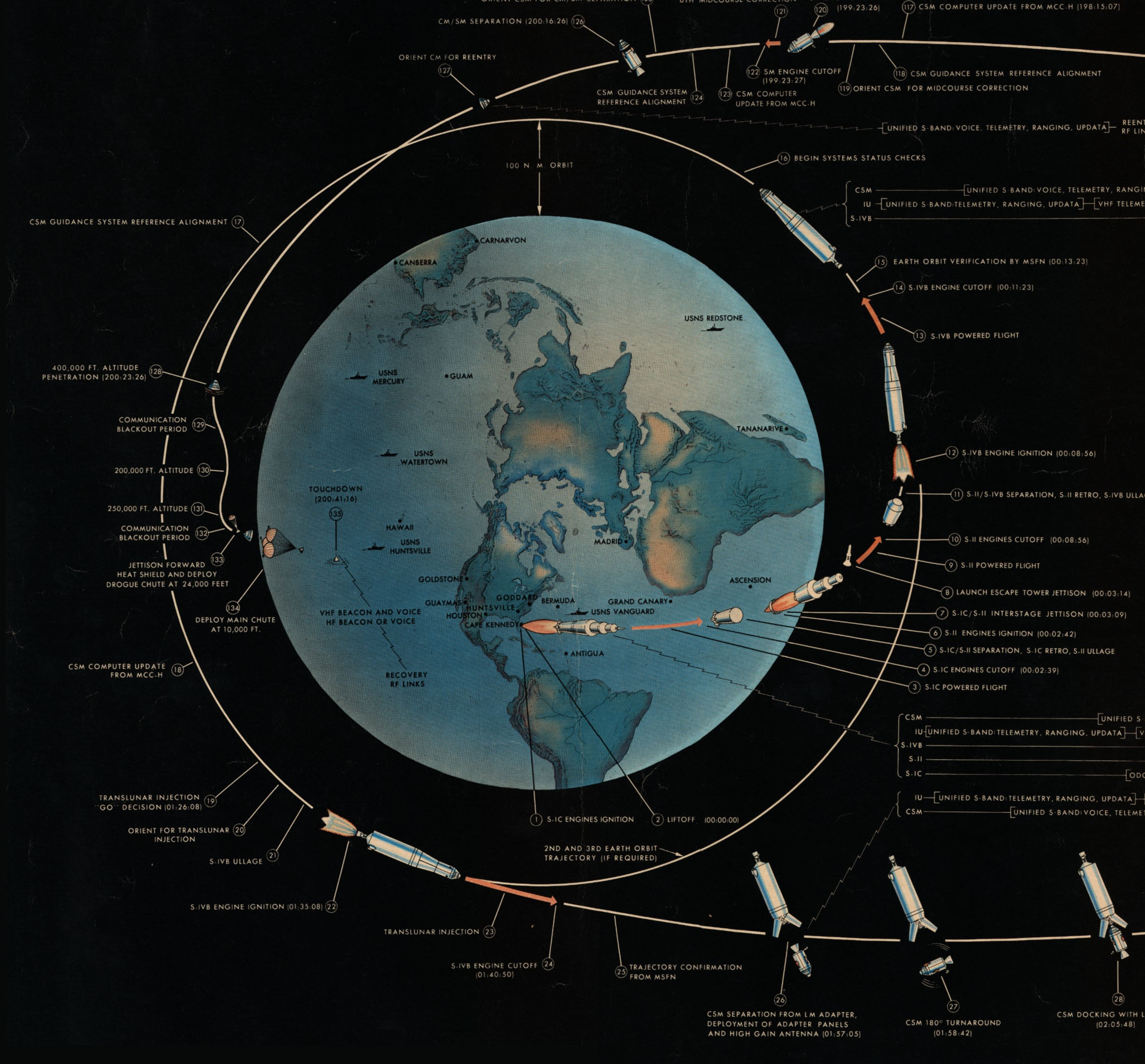# Numbers

Part of:Apollo Mission Flight Plan - 1967 Credit: NASA Jump down to Career Profiles

Astronomers use numbers all the time (especially really big ones!). Astronomy grew out of solving problems about time and distance. Problems like: measuring the distances to stars, working out how bright stars are, locating objects using angles and coordinates. Many famous astronomers were experts in maths as well as astronomy.

If you are interested in maths then you may be interested in a career as a theoretical astrophysicist. Dealing with complicated equations to guide observational astronomers in what they need to test, to answer the big questions. You might also want to explore careers in space agencies working out the trajectories of rockets and space probes. Most jobs related to astronomy have some maths involved, but these are examples which have a stronger maths component.

Astrophysicists apply their knowledge of maths to solve problems about the Universe. They collect information using telescopes, and use maths and statistics to interpret the information. Astrophysicists also use mathematical models and formulas to understand the physics of the Universe. We would not have been able to discover black holes or know that the Universe is expanding without maths.

The recent detection of gravitational waves is exciting because it gives astronomers a new way of looking at the Universe. Physicists are applying maths in new ways to understand gravitational waves and the objects that create them.

If you choose to study maths at university, you can move into a career in astronomy later (or just carry on with it as a hobby!). If you study astronomy or astrophysics at university, you will leave with strong maths skills. Lots of different employers value these skills.

In the career profiles on this page you can find out more about astronomers who were also interested in numbers: